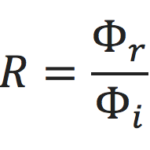# What We Measure, Part 1: Reflectance

The material properties we measure are reflectance and transmittance, or more typically, reflectance factor and transmittance factor. Transmittance and reflectance are the more general measurements, but as we will see below, when we compare a sample and a physical reference standard, measured under a specific optical configuration, the proper terms are "transmittance factor" and "reflectance factor." The specific definitions can be complex and confusing, but we will present an explanation at the level of the non-expert in the spectroscopy and color measurement communities. Reflectance is the more complex measurement, and this post will contain technical details explaining what it is. Measuring transmittance is a simpler procedure, but it comes with its own set of complications that we will cover in turn.

So, reflectance is simply a ratio: light reflecting off an object divided by the light incident on the object. We use Φ to indicate the amount of light, the subscripts r and i for reflected and incident light. Sorry for the math, but a little bit is going to be required:What’s so complicated about that? In principle, nothing, but consider this graphic showing one arrangement of light source, sample and detector. Since this diagram shows a specific optical arrangement, this measurement is properly referred to as "reflectance factor." We show a beam of white light incident on the blue sample, and a beam of blue light reflecting off, and we position a detector to measure the reflected light. The problem is that besides measuring the reflected light — the numerator of the equation — we also need to measure the incident light for the denominator. That would require positioning the detector where the sample is, or someplace else in the optical path. For higher-end or research instruments this is often possible, but for most devices this is not practical and would be too expensive to enable.

How then do we measure reflectance, when the vast majority of instruments cannot even measure the simple values in our reflectance equation? We need some help from others. Usually that help comes from the manufacturer of the instrument. In any case, what we need is an object that is of known reflectance. The measurement community has a special word for such samples: standards; in this case reference standards.

Imagine you are trying to make accurate voltage readings. You purchase a battery that always produces exactly 5V.‡ In the morning, you hook up this perfect battery and adjust the voltmeter so it reads 5V. An advanced voltmeter might also have the ability to dial in 0V. Between these two calibration steps, your meter can now accurately measure voltage. When we do the equivalent of the 5V and 0V measurements in our calibration procedure, we are measuring the reference standard and a black standard. The final form of reflectance is this:Here Φd is the dark measurement and the reference reflectance data Rs.

In a real instrument, we cannot measure light quantities directly, but we infer the amount of light from the detector signal. This signal (current or voltage) is digitized and the processed in the control software.

The above measurement is carried out at every point in the spectrum. Depending on the application, this could be 250nm to 2500nm sampled every 1nm or 400nm to 700nm sampled every 10nm. Or mid-IR all the way out to 20µ. And from the reflectance spectrum, derivative values can be calculated, such as color, whiteness, and more. Or you can infer chemical composition, fading, and even counterfeit products! But that is another column...

‡ You may well ask, “how close to 5V does it have to be for me to say exactly 5V?” Good question. That depends on the uncertainty of the measurement process. We will cover that in our discussion about Traceability.